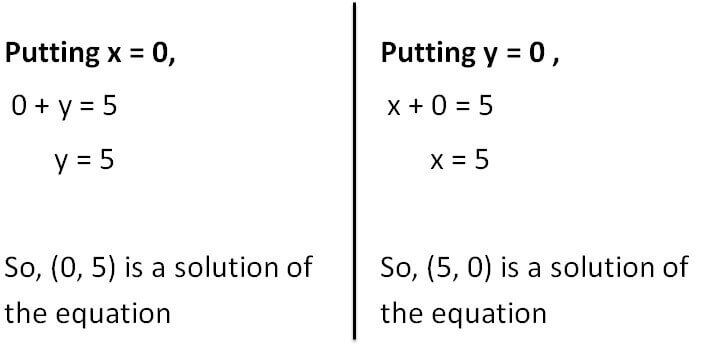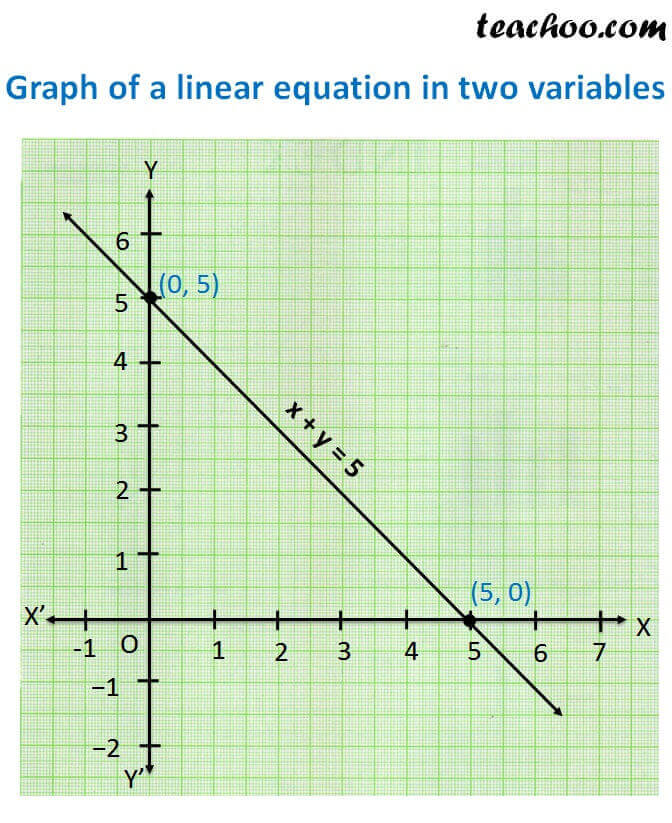Graph of linear equations

Chapter 4 Class 9 Linear Equations in Two Variables
Concept wise

Let us draw the graph of

x + y = 5

To draw the graph, we need at least two solutions of the equation.

Finding solutionsPlotting points.

 x 0 5 y 5 0Learn in your speed, with individual attention - Teachoo Maths 1-on-1 Class